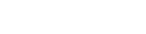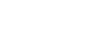# JavaScript Date

The Date object in JavaScript allows you to interact with dates and times, such as days, months, years, hours, minutes, seconds, and milliseconds. To acquire a Date for the current time or Date, use new Date(). The time is maintained internally as a number of milliseconds since January 1, 1970 UTC. Lets take an example to fetch current date and time.

``````
const x = new Date();
let val = x.getDate();
console.log(val); // 18

``````
MethodDescriptionExample
getDate()The day of the month is returned.
``````
const x = new Date();
let val = x.getDate();
console.log(val); // 18

``````
getDay()The day of the week is returned.
``````
const x = new Date();
let val = x.getDay();
console.log(val); // 6

``````
getFullYear()Returns the year
``````
const x = new Date();
let val = x.getFullYear();
console.log(val); // 2022

``````
getHours()Returns the hour
``````
const x = new Date();
let val = x.getHours();
console.log(val); // 20

``````
getMinutes()Returns the minute
``````
const x = new Date();
let val = x.getMinutes();
console.log(val); // 13

``````
getSeconds()Returns the seconds
``````
const x = new Date();
let val = x.getSeconds();
console.log(val); // 40

``````
getMilliseconds()Returns the milliseconds
``````
const x = new Date();
let val = x.getMilliseconds();
console.log(val); // 277

``````
setTime()Sets a date to a number of milliseconds
``````
const x = new Date();
x.setTime(1647649574758);
console.log(x); // 2022-03-19T00:26:14.758Z

``````
setDate()Sets a date
``````
const x = new Date();
x.setDate(11);
console.log(x); // 2022-03-11T01:12:22.774Z

``````
toString()This function converts a Date object to a string.
``````
const d = new Date();
let val = x.toString();
console.log(typeof val); //string

``````
parse()Returns the value of milliseconds since January 1, 1970 after parsing a date string.
``````
let val = Date.parse("April 17, 1992");
console.log(val); //703468800000

``````